Search by Topic

Resources tagged with Addition & subtraction similar to Christmas Presents:

Filter by: Content type:
Age range:
Challenge level:

There are 215 resultsPrompt Cards

Age 7 to 11 Challenge Level:

These two group activities use mathematical reasoning - one is numerical, one geometric.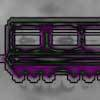Train Carriages

Age 5 to 11 Challenge Level:

Suppose there is a train with 24 carriages which are going to be put together to make up some new trains. Can you find all the ways that this can be done?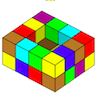Painting Possibilities

Age 7 to 11 Challenge Level:

This task, written for the National Young Mathematicians' Award 2016, involves open-topped boxes made with interlocking cubes. Explore the number of units of paint that are needed to cover the boxes. . . .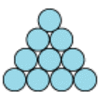Difference

Age 7 to 11 Challenge Level:

Place the numbers 1 to 10 in the circles so that each number is the difference between the two numbers just below it.Code Breaker

Age 7 to 11 Challenge Level:

This problem is based on a code using two different prime numbers less than 10. You'll need to multiply them together and shift the alphabet forwards by the result. Can you decipher the code?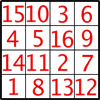Magic Constants

Age 7 to 11 Challenge Level:

In a Magic Square all the rows, columns and diagonals add to the 'Magic Constant'. How would you change the magic constant of this square?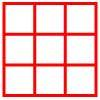Age 5 to 11 Challenge Level:

Place six toy ladybirds into the box so that there are two ladybirds in every column and every row.A Square of Numbers

Age 7 to 11 Challenge Level:

Can you put the numbers 1 to 8 into the circles so that the four calculations are correct?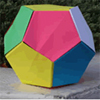Dodecamagic

Age 7 to 11 Challenge Level:

Here you see the front and back views of a dodecahedron. Each vertex has been numbered so that the numbers around each pentagonal face add up to 65. Can you find all the missing numbers?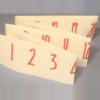Folded Number Line

Age 7 to 11 Challenge Level:

When I fold a 0-20 number line, I end up with 'stacks' of numbers on top of each other. These challenges involve varying the length of the number line and investigating the 'stack totals'.Worms

Age 7 to 11 Challenge Level:

Place this "worm" on the 100 square and find the total of the four squares it covers. Keeping its head in the same place, what other totals can you make?Plants

Age 5 to 11 Challenge Level:

Three children are going to buy some plants for their birthdays. They will plant them within circular paths. How could they do this?Calendar Calculations

Age 7 to 11 Challenge Level:

Try adding together the dates of all the days in one week. Now multiply the first date by 7 and add 21. Can you explain what happens?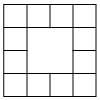Prison Cells

Age 7 to 11 Challenge Level:

There are 78 prisoners in a square cell block of twelve cells. The clever prison warder arranged them so there were 25 along each wall of the prison block. How did he do it?Page Numbers

Age 7 to 11 Short Challenge Level:

Exactly 195 digits have been used to number the pages in a book. How many pages does the book have?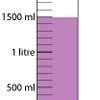Oh! Harry!

Age 7 to 11 Challenge Level:

A group of children are using measuring cylinders but they lose the labels. Can you help relabel them?Spell by Numbers

Age 7 to 11 Challenge Level:

Can you substitute numbers for the letters in these sums?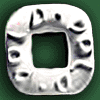Polo Square

Age 7 to 11 Challenge Level:

Arrange eight of the numbers between 1 and 9 in the Polo Square below so that each side adds to the same total.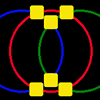Magic Circles

Age 7 to 11 Challenge Level:

Put the numbers 1, 2, 3, 4, 5, 6 into the squares so that the numbers on each circle add up to the same amount. Can you find the rule for giving another set of six numbers?Arranging the Tables

Age 7 to 11 Challenge Level:

There are 44 people coming to a dinner party. There are 15 square tables that seat 4 people. Find a way to seat the 44 people using all 15 tables, with no empty places.Zargon Glasses

Age 7 to 11 Challenge Level:

Zumf makes spectacles for the residents of the planet Zargon, who have either 3 eyes or 4 eyes. How many lenses will Zumf need to make all the different orders for 9 families?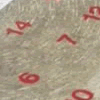All Seated

Age 7 to 11 Challenge Level:

Look carefully at the numbers. What do you notice? Can you make another square using the numbers 1 to 16, that displays the same properties?Abundant Numbers

Age 7 to 11 Challenge Level:

48 is called an abundant number because it is less than the sum of its factors (without itself). Can you find some more abundant numbers?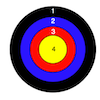Dart Target

Age 7 to 11 Challenge Level:

This task, written for the National Young Mathematicians' Award 2016, invites you to explore the different combinations of scores that you might get on these dart boards.Age 5 to 11 Challenge Level:

Try out this number trick. What happens with different starting numbers? What do you notice?A-magical Number Maze

Age 7 to 11 Challenge Level:

This magic square has operations written in it, to make it into a maze. Start wherever you like, go through every cell and go out a total of 15!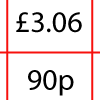How Much Did it Cost?

Age 7 to 11 Challenge Level:

Use your logical-thinking skills to deduce how much Dan's crisps and ice-cream cost altogether.Sometimes We Lose Things

Age 7 to 11 Challenge Level:

Well now, what would happen if we lost all the nines in our number system? Have a go at writing the numbers out in this way and have a look at the multiplications table.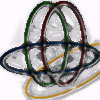Bean Bags for Bernard's Bag

Age 7 to 11 Challenge Level:

How could you put eight beanbags in the hoops so that there are four in the blue hoop, five in the red and six in the yellow? Can you find all the ways of doing this?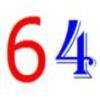Age 7 to 11 Challenge Level:

Write the numbers up to 64 in an interesting way so that the shape they make at the end is interesting, different, more exciting ... than just a square.A Mixed-up Clock

Age 7 to 11 Challenge Level:

There is a clock-face where the numbers have become all mixed up. Can you find out where all the numbers have got to from these ten statements?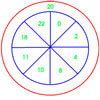On Target

Age 7 to 11 Challenge Level:

You have 5 darts and your target score is 44. How many different ways could you score 44?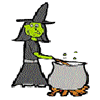Hubble, Bubble

Age 7 to 11 Challenge Level:

Winifred Wytsh bought a box each of jelly babies, milk jelly bears, yellow jelly bees and jelly belly beans. In how many different ways could she make a jolly jelly feast with 32 legs?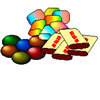The Puzzling Sweet Shop

Age 7 to 11 Challenge Level:

There were chews for 2p, mini eggs for 3p, Chocko bars for 5p and lollypops for 7p in the sweet shop. What could each of the children buy with their money?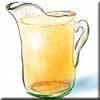Pouring the Punch Drink

Age 7 to 11 Challenge Level:

There are 4 jugs which hold 9 litres, 7 litres, 4 litres and 2 litres. Find a way to pour 9 litres of drink from one jug to another until you are left with exactly 3 litres in three of the jugs.The Pied Piper of Hamelin

Age 7 to 11 Challenge Level:

This problem is based on the story of the Pied Piper of Hamelin. Investigate the different numbers of people and rats there could have been if you know how many legs there are altogether!Being Resourceful - Primary Number

Age 5 to 11 Challenge Level:

Number problems at primary level that require careful consideration.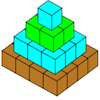Open Squares

Age 7 to 11 Challenge Level:

This task, written for the National Young Mathematicians' Award 2016, focuses on 'open squares'. What would the next five open squares look like?Cubes Within Cubes

Age 7 to 14 Challenge Level:

We start with one yellow cube and build around it to make a 3x3x3 cube with red cubes. Then we build around that red cube with blue cubes and so on. How many cubes of each colour have we used?Two Primes Make One Square

Age 7 to 11 Challenge Level:

Can you make square numbers by adding two prime numbers together?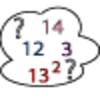Seven Square Numbers

Age 7 to 11 Challenge Level:

Add the sum of the squares of four numbers between 10 and 20 to the sum of the squares of three numbers less than 6 to make the square of another, larger, number.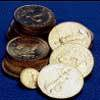Five Coins

Age 7 to 11 Challenge Level:

Ben has five coins in his pocket. How much money might he have?Street Sequences

Age 5 to 11 Challenge Level:

Investigate what happens when you add house numbers along a street in different ways.Age 7 to 11 Challenge Level:

Can you put plus signs in so this is true? 1 2 3 4 5 6 7 8 9 = 99 How many ways can you do it?Rabbits in the Pen

Age 7 to 11 Challenge Level:

Using the statements, can you work out how many of each type of rabbit there are in these pens?Clocked

Age 11 to 14 Challenge Level:

Is it possible to rearrange the numbers 1,2......12 around a clock face in such a way that every two numbers in adjacent positions differ by any of 3, 4 or 5 hours?Oddly

Age 7 to 11 Challenge Level:

Find the sum of all three-digit numbers each of whose digits is odd.Sums and Differences 2

Age 7 to 11 Challenge Level:

Find the sum and difference between a pair of two-digit numbers. Now find the sum and difference between the sum and difference! What happens?Sticky Dice

Age 7 to 11 Challenge Level:

Throughout these challenges, the touching faces of any adjacent dice must have the same number. Can you find a way of making the total on the top come to each number from 11 to 18 inclusive?More Children and Plants

Age 7 to 14 Challenge Level:

This challenge extends the Plants investigation so now four or more children are involved.